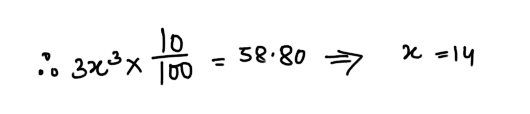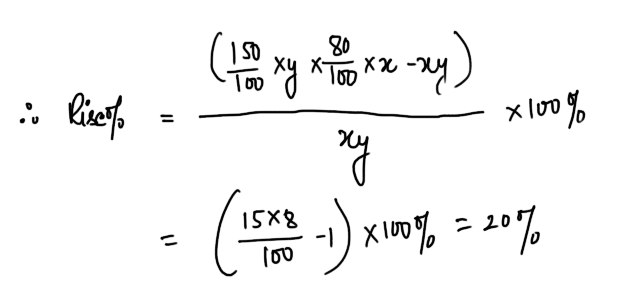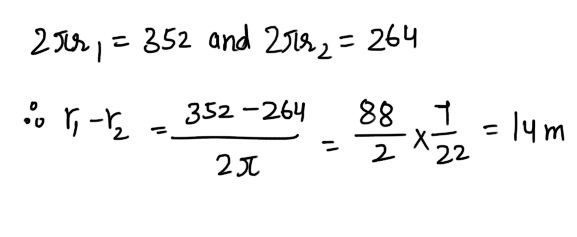# Mixed Aptitude Quiz for SBI Clerk Mains (Part - 12)#### Ques 1.

A man bought 300 guavas at 10 a rupee and 300 more at 15 a rupee and sold them at 25 for 2 rupees. How much did he lose ?
(a) Rs 2
(b) Rs 3.
(c) Insufficient data
(d) None of these
Ans 1.#### Ques 2.

A can make a table in 6 days and B two tables in 16 days. Hoe long will they take to make sixty three tables working together ?
(a) 216 days
(b) 196 days
(c) 189 days
(d) 252 days
Ans 2.#### Ques 3.

A certain number of boys spent thrice as many ten paise coins, as the cube of the number of 58.80. How many boys were there?
(a) 14
(b) 7
(c) 28
(d) None of these
Ans 3. Let the number of boys be x.#### Ques 4.

An amount doubles itself in 8 years at simple interest. At the same rate it will become 3 times in
(a) 12 years
(b) 16 years
(c) 18 years
(d) 24 years
Ans 4.#### Ques 5.

When the sale price of an item was increased by 50%, there was a shortall of 20% in his sales. What is the percentage effect on the revenue from sales ?
(a) 15 % rise
(b) 15% fall
(c) 20% rise
(d) 20 Fall
Ans 5. Let the number of goods sold previously be x and the sale price per piece be y.#### Ques 6.

A watch gains 4 seconds per hour.What time will it show at 8 a.m. on 22nd of a month if it is set right at 1 p.m. on 20th of the same month ?
(a) 8 hr. 2 min. 40 sec.
(b) 8 hr. 3 min. 20 sec.
(c) 8 hr. 2 min. 52 sec.
(d) 8 hr. 2 min. 45 sec.
Ans 6.  Time interval = ( 19 + 24) hours = 43 hours
= 43 x 4 seconds = 172 s
= 2 minutes 52 seconds
Hence, the clock would show 8 hr. 2 min. 52 sec.

#### Ques 7.

To fill a cistern, pipes A, B and C take 20 minutes, 15 minutes and 12 minutes respectively . The time that the three pipes together will take to fill the cistern is
(a) 5 min.
(b) 7 min.
(c) 10 min.
(d) 12 min.
Ans 7.#### Ques 8.

The average weight of A, B , C and D is 67 kg.When a fifth man E joins then the average weight is reduced by 2 kg. A is replaced by F whose weight i more than E's by 4 kg. This brought down the average weight to 64 kg. The weight of A is
(a) 68 kg
(b) 73 kg
(c) 72 Kg
(d) 55 kg
Ans 8. weight of A + B + C + D = ( 4 x 67) kg
=268 kg
Weight of A + B + C + D + E = ( 5 x 65 ) kg
= 325 kg
Weight of E = 325- 268 = 57 kg
Weight of F = 57 + 4 = 61 kg
Weight of B + C + D + F = ( 4 x 64)
= 256kg
or Weight of  B + C + D = 256-61
= 195 kg
. . Weight of A = 268- 195 = 73 kg

#### Ques 9.

The circumferences of two concentric cricles are 352 ,m and 264 m respectively . The difference between the radii of the circles is
(a) 7 m
(b) 10 m
(c) 14 m
(d) 15 m
Ans 9.